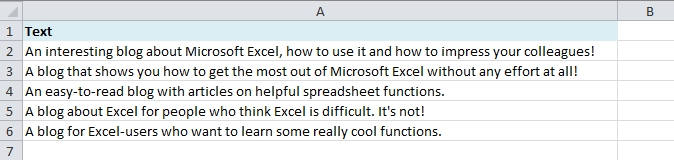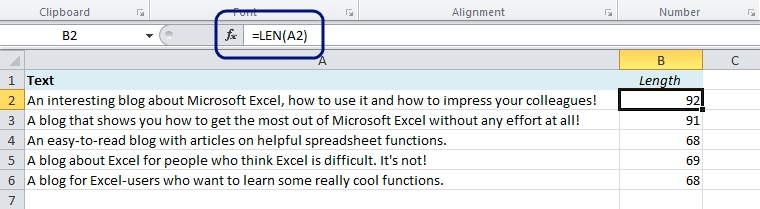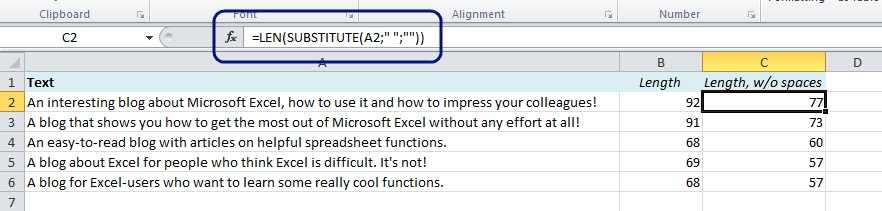# How to use Excel to Count Characters in a Text String

We often need to measure the length of a text, for example if we write a text that will appear on a website. Typically, in a web shop the product introduction text has to be of a certain length in order to fit within the frames.

Of course, the easiest way to do this is in Excel. This is extremely easy – it only takes a few seconds:

1. Import the text into Excel, or type it:2. Use the LEN formula:

To determine the length of the text in A2, use this formula:

=LEN(A2)

Copy down.## Bonus trick: How to exclude spaces when you count characters in Excel

Use the SUBSTITUTE function to remove the spaces: =SUBSTITUTE(A2,” “,””) and wrap it inside the LEN function:

=LEN(SUBSTITUTE(A2,” “,””))

Explanation: The SUBSTITUTE function substitutes the spaces, ” “, with nothing, “”.Other easy tricks:

Are you using a non-English version of Excel? Click here for translations of the 100 most common functions.